Learn about smartphone sensors in the next Xcel masterclass with Tinkerbee CEO, Anupam! Learn about smartphone sensors in the next Xcel masterclass with Tinkerbee CEO, Anupam!

# Andhra Pradesh SSC Board Question Paper for Class 10th Maths Paper 1 2015 In PDF

## AP SSC or 10th Class Question Paper Mathematics Paper 1 English Medium 2015 with Solutions – Free Download

Andhra Pradesh SSC (Class 10) Maths 2015 question paper 1 with solutions are provided here in a downloadable pdf format and also in the text so that the students can easily access them. Along with the solutions, they can also get the Maths question paper 1 2015 Class 10 SSC for reference. Students are able to access all the Andhra Pradesh board previous year maths question papers here. AP 10th Class Mathematics Question Paper 2015 Paper 1 can be easily downloaded in PDF format. Students can practice this Andhra Pradesh SSC 10th Class Maths 2015 question paper 1 solutions and verify the answers provided by BYJU’S. Solving 2015 Maths question paper 1 for Class 10 will help the students to predict what type of questions will appear in the exam.

## Download AP SSC 2015 Question Paper Maths Paper 1 With Solutions :-

### QUESTION PAPER CODE 15E(A)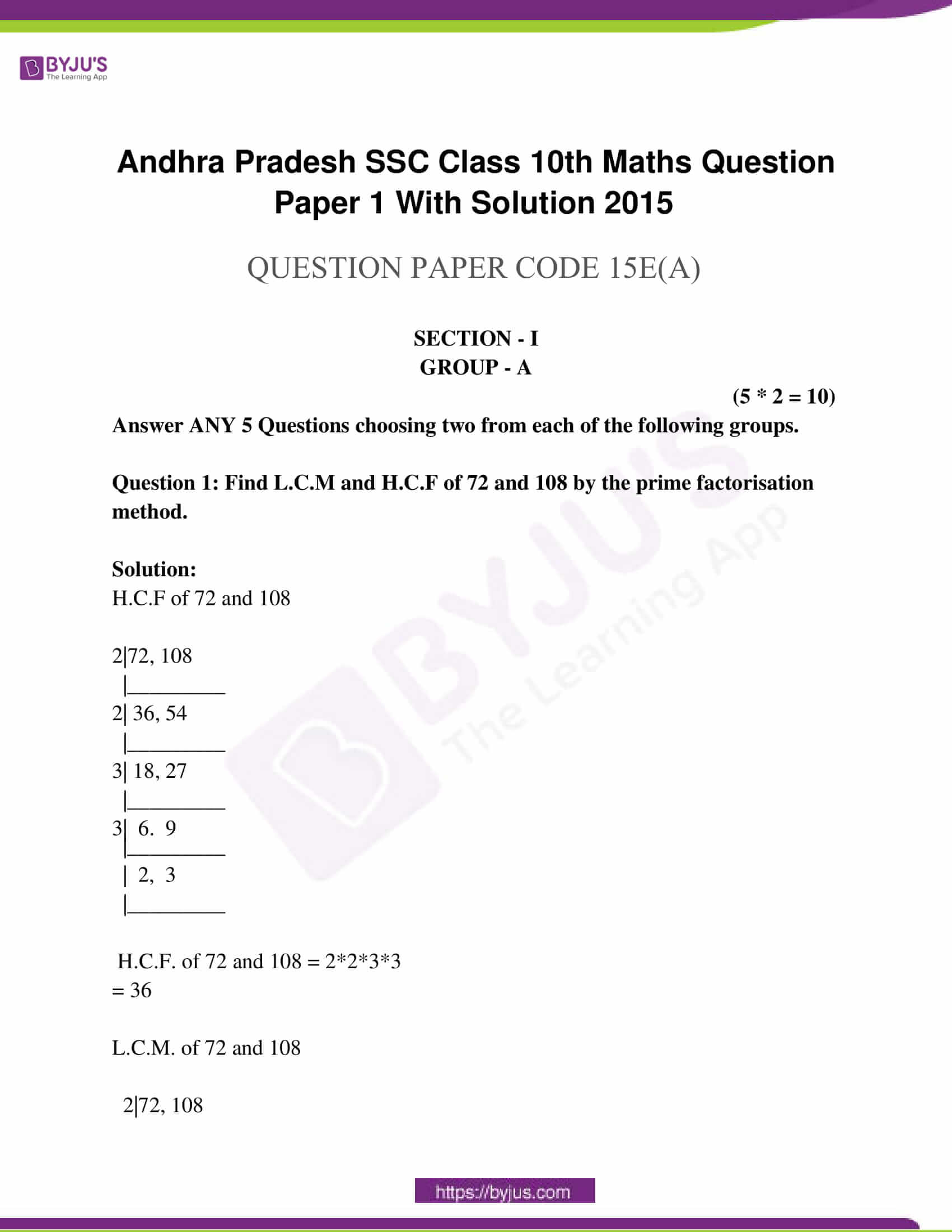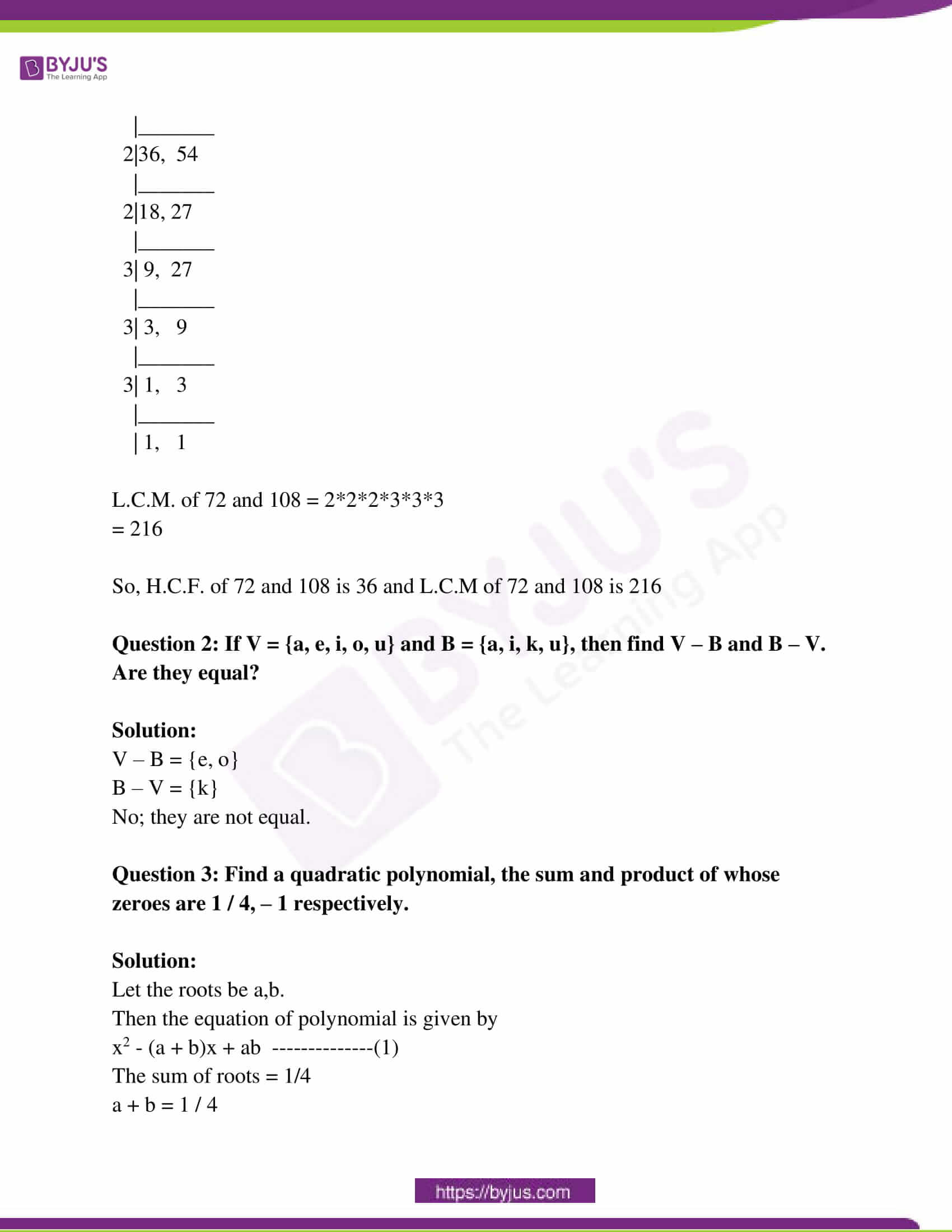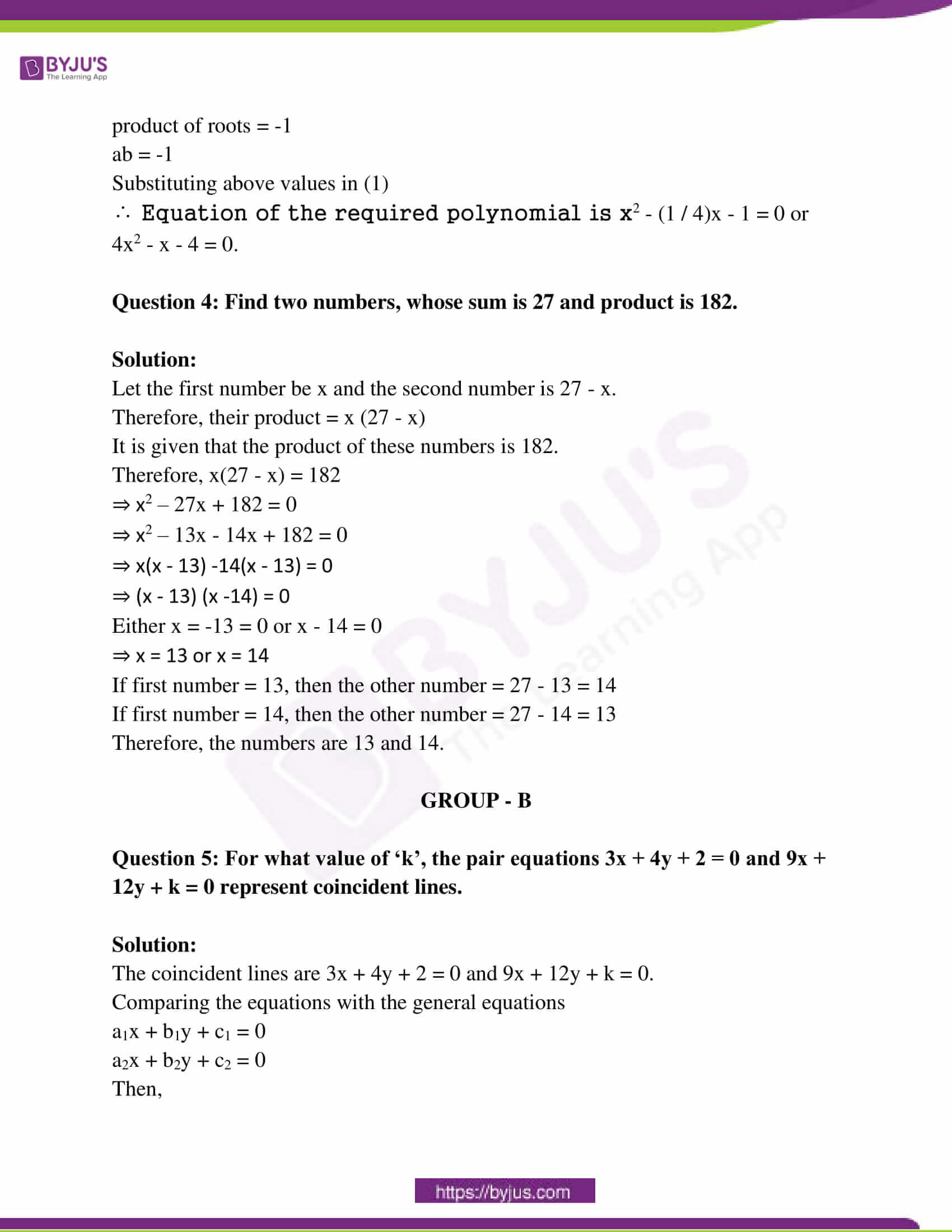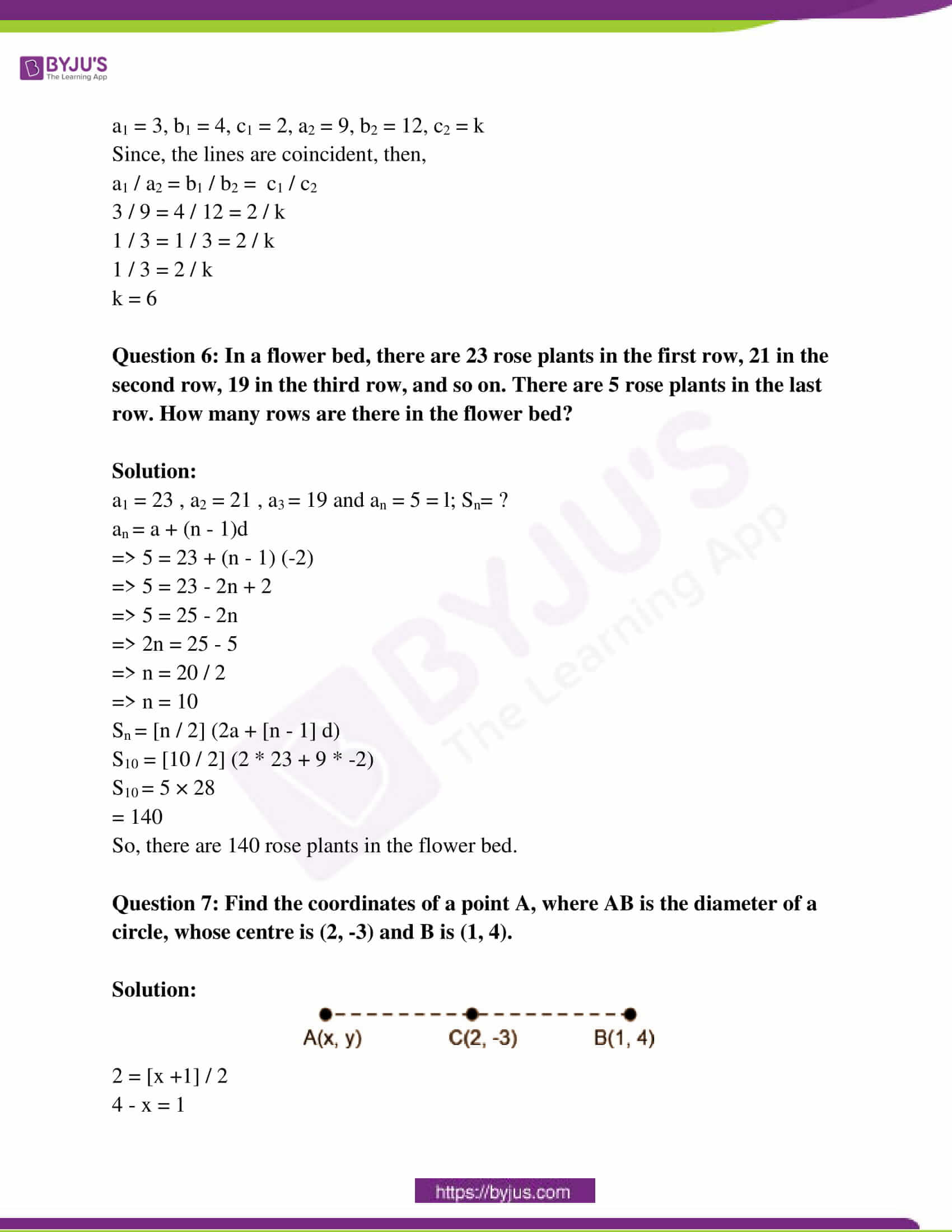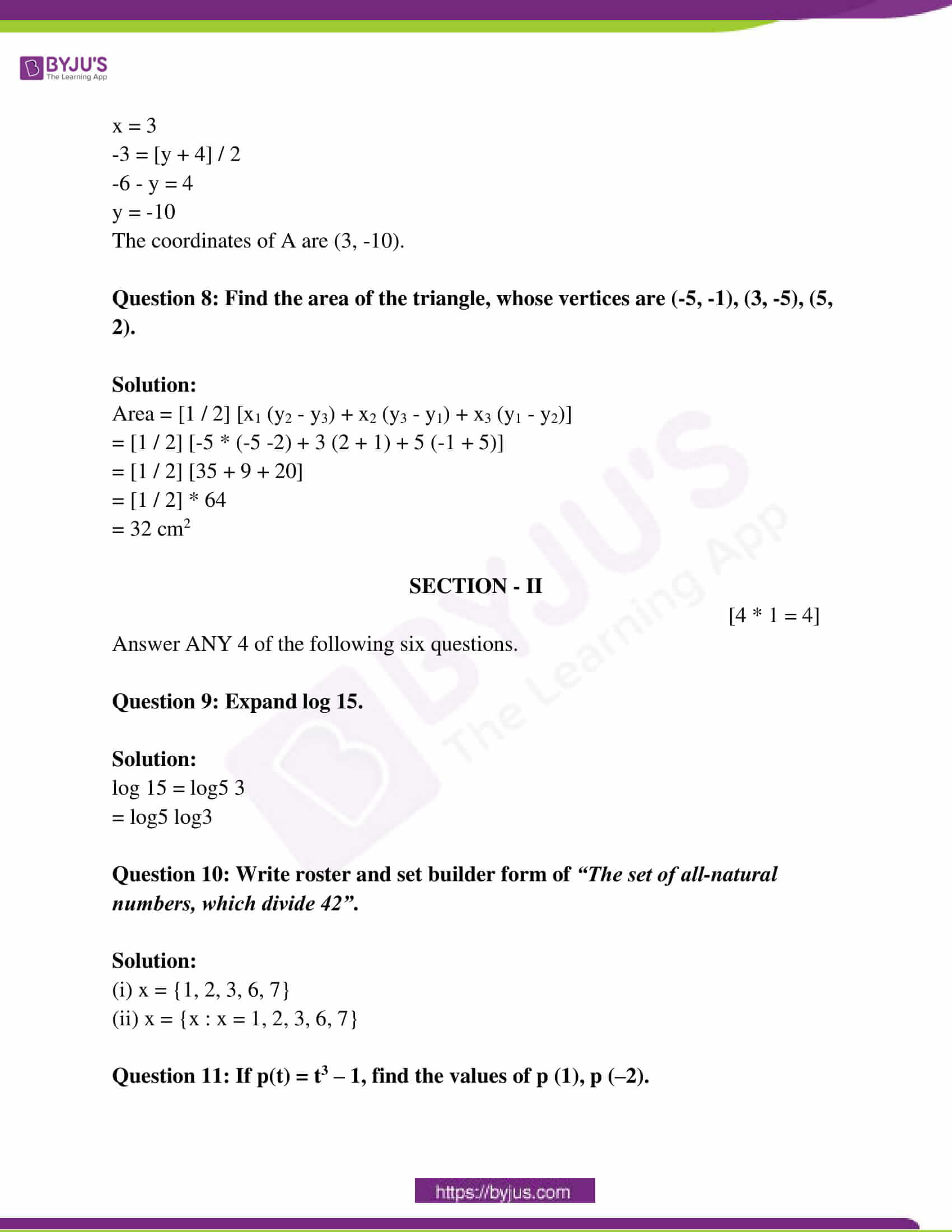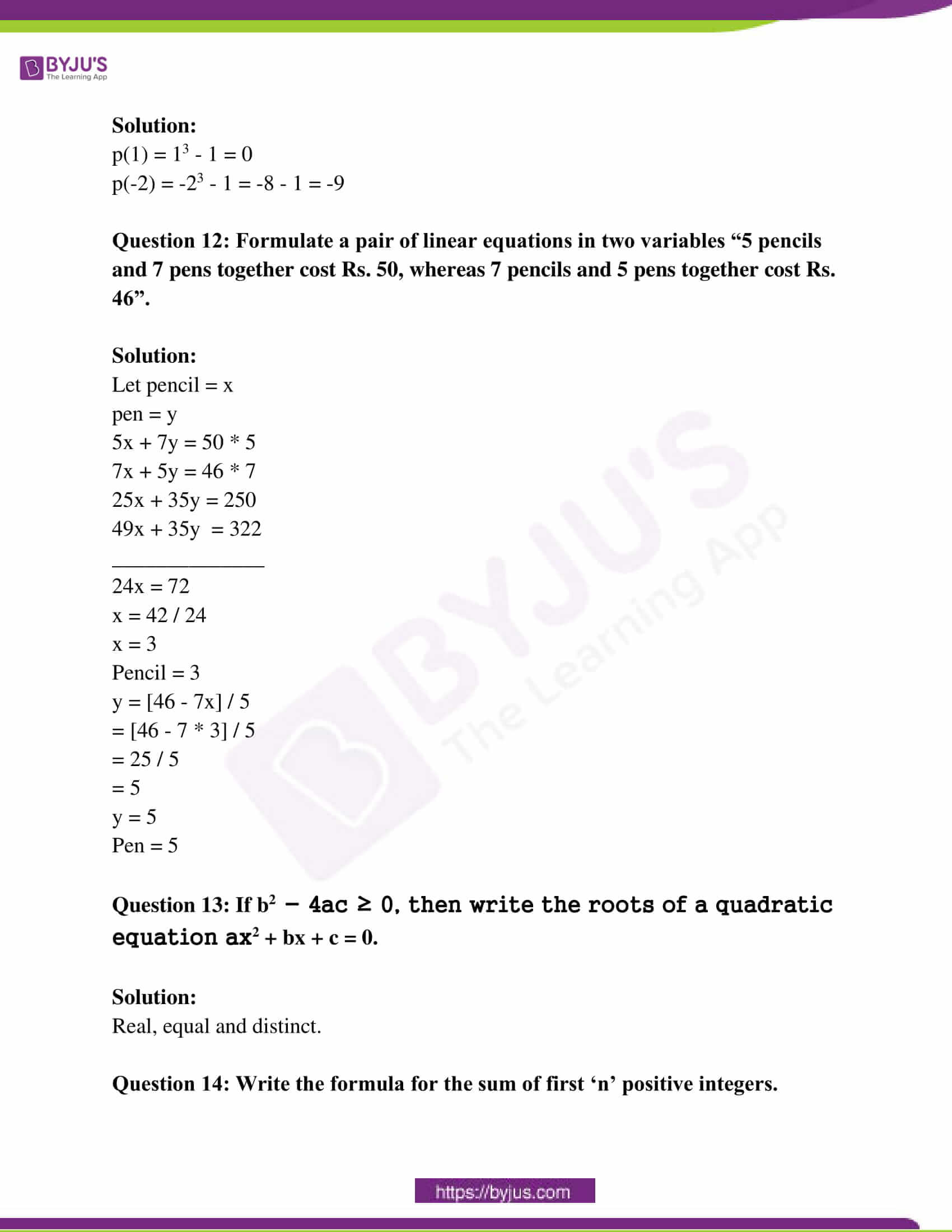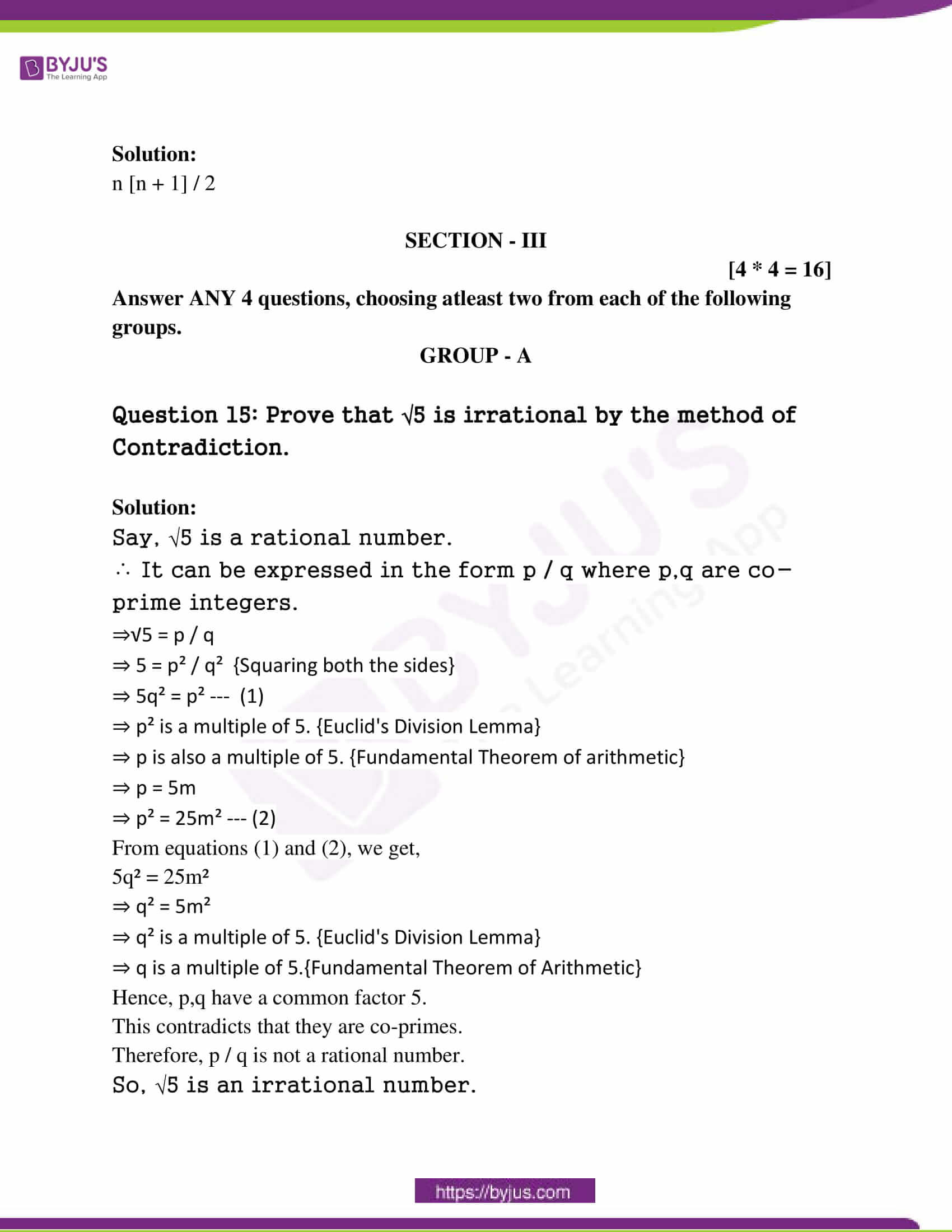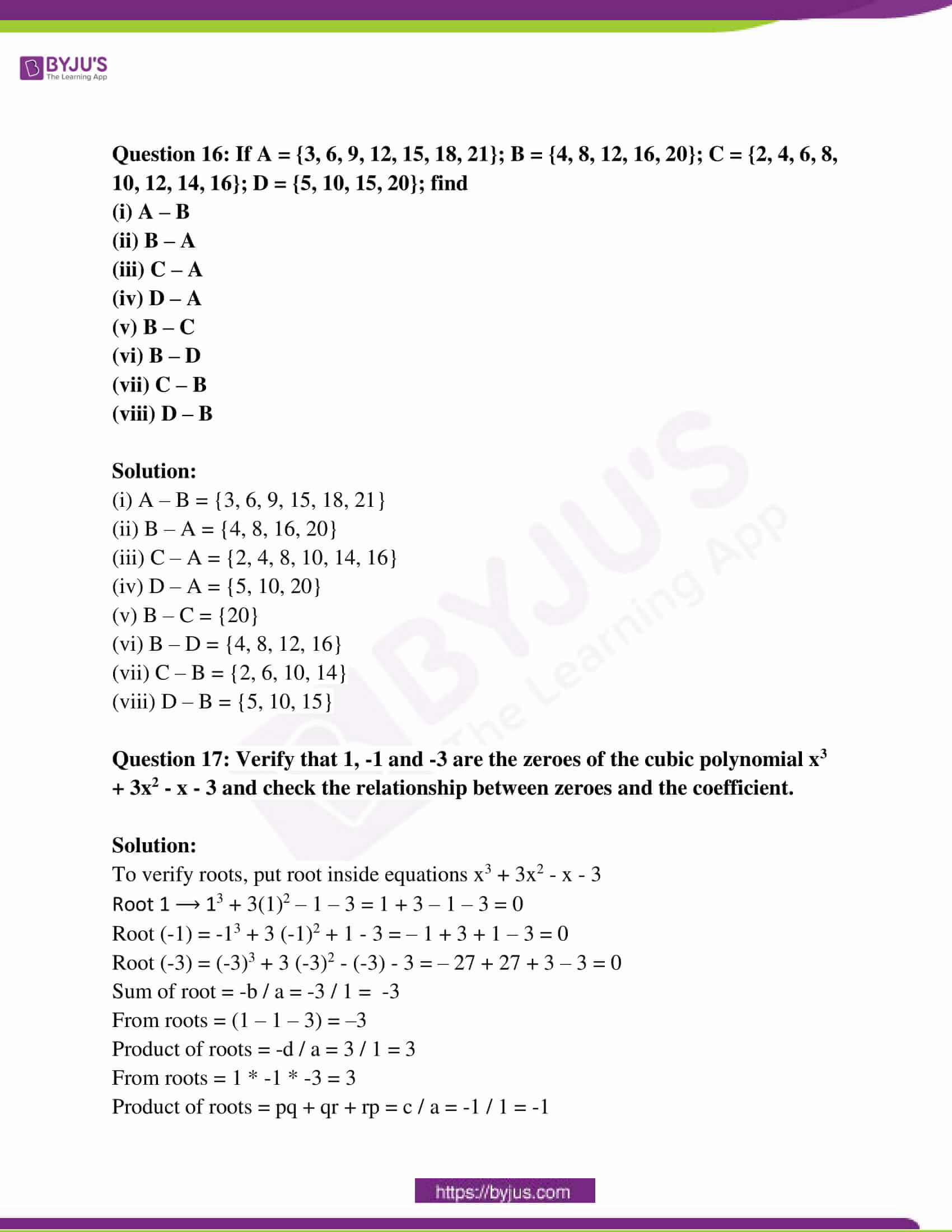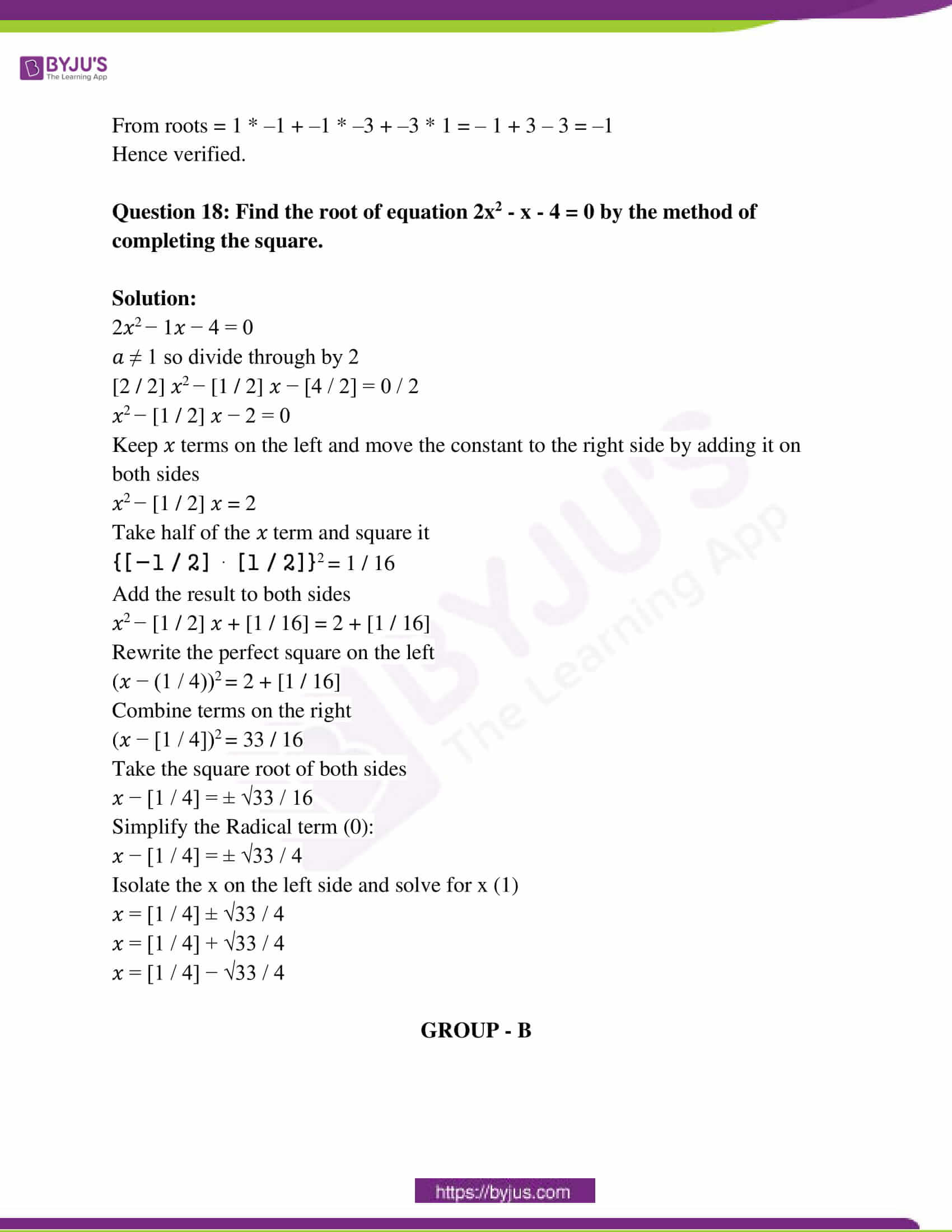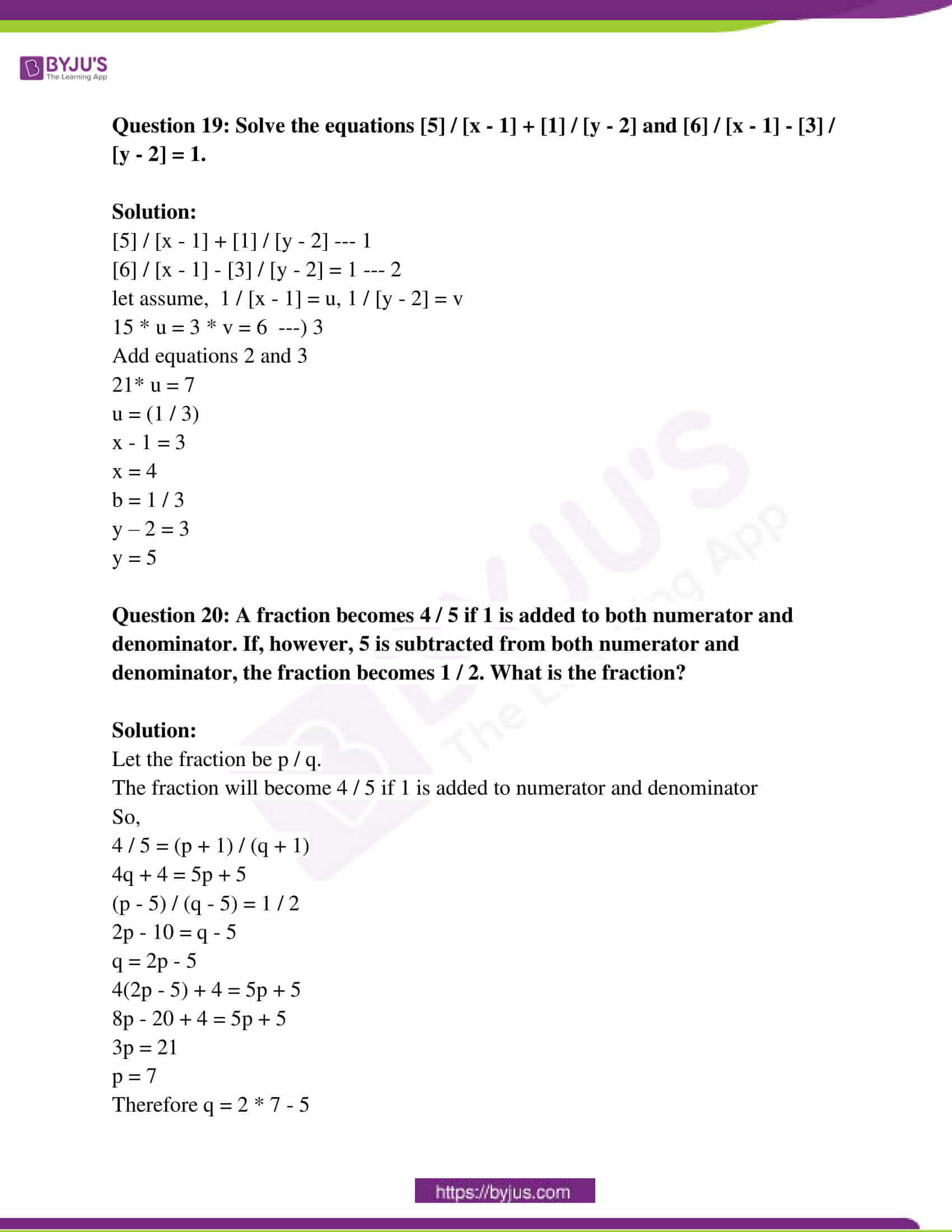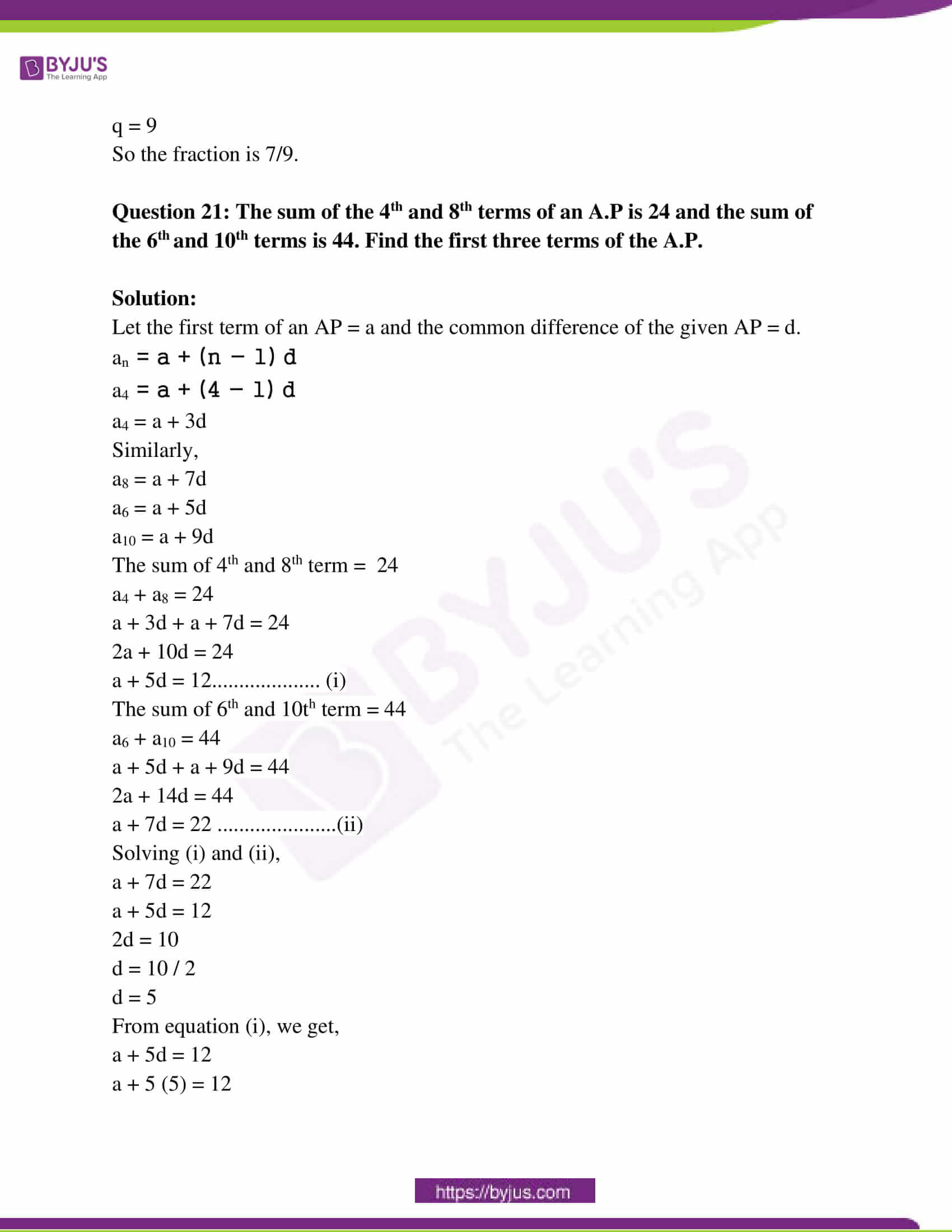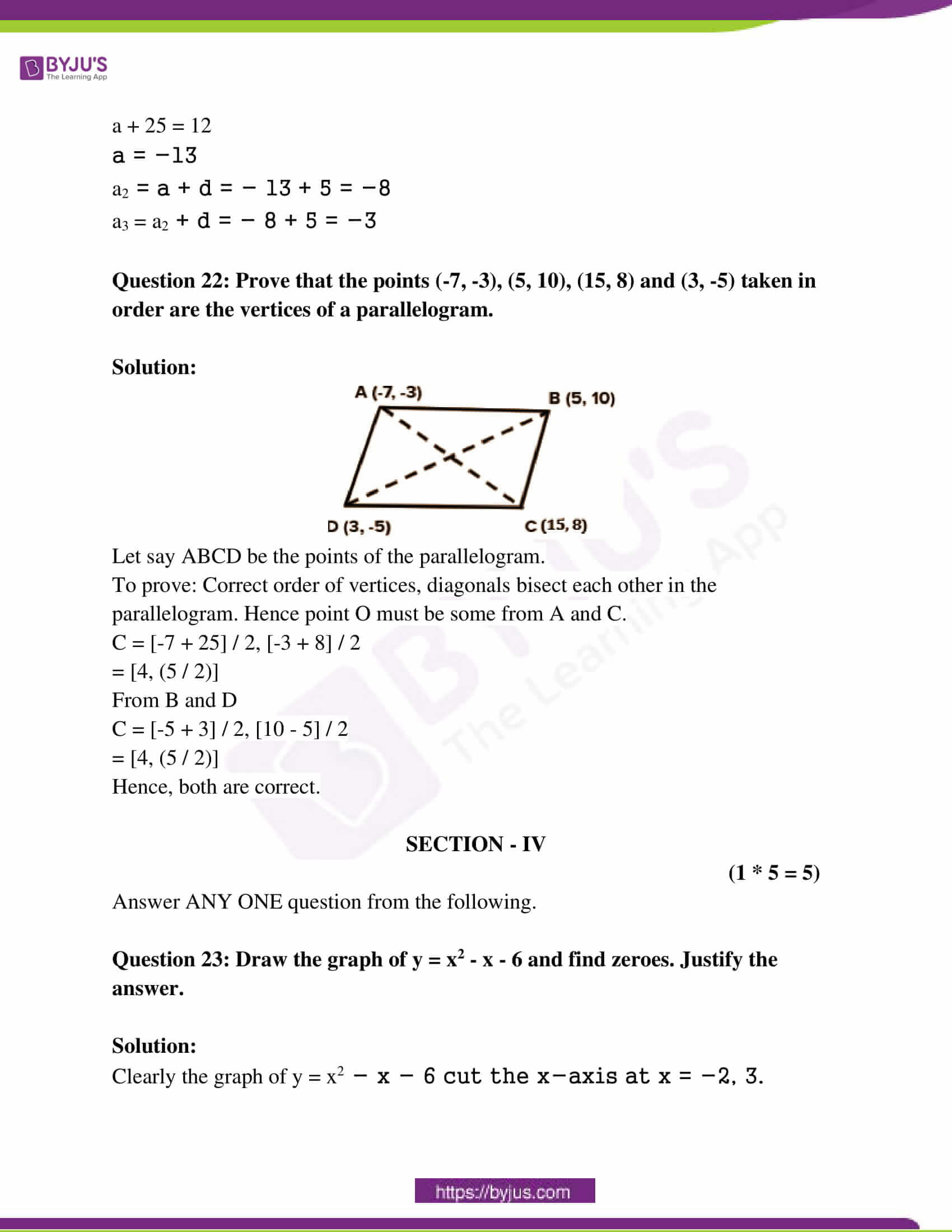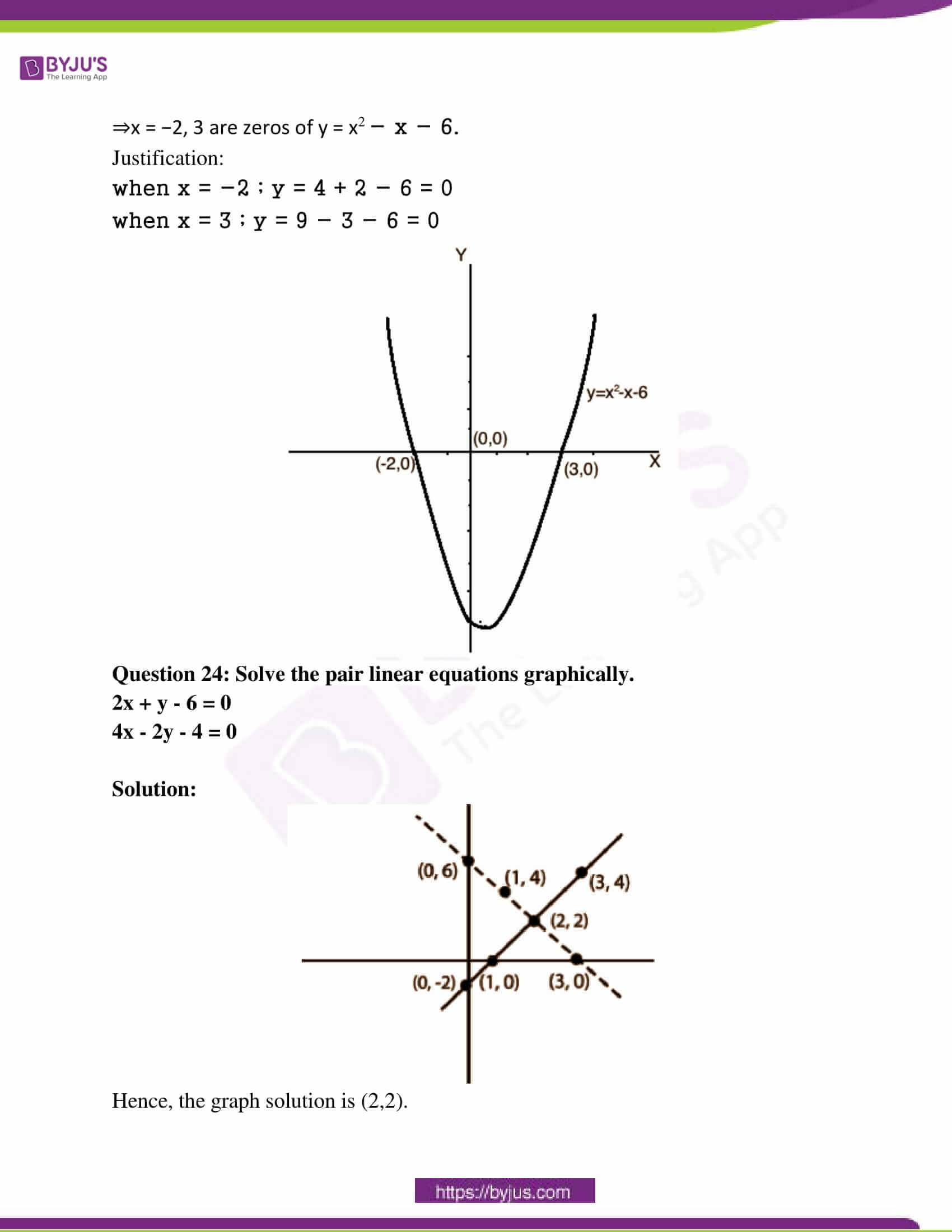SECTION – I

GROUP – A

(5 * 2 = 10)

Answer ANY 5 Questions choosing two from each of the following groups.

Question 1: Find L.C.M and H.C.F of 72 and 108 by the prime factorisation method.

Solution:

H.C.F of 72 and 108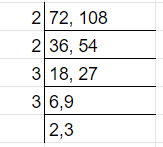H.C.F. of 72 and 108 = 2*2*3*3

= 36

L.C.M. of 72 and 108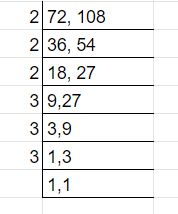L.C.M. of 72 and 108 = 2*2*2*3*3*3

= 216

So, H.C.F. of 72 and 108 is 36 and L.C.M of 72 and 108 is 216

Question 2: If V = {a, e, i, o, u} and B = {a, i, k, u}, then find V – B and B – V. Are they equal?

Solution:

V – B = {e, o}

B – V = {k}

No; they are not equal.

Question 3: Find a quadratic polynomial, the sum and product of whose zeroes are 1 / 4, – 1 respectively.

Solution:

Let the roots be a,b.

Then the equation of polynomial is given by

x2 – (a + b)x + ab ————–(1)

The sum of roots = 1/4

a + b = 1 / 4

product of roots = -1

ab = -1

Substituting above values in (1)

∴ Equation of the required polynomial is x2 – (1 / 4)x – 1 = 0 or 4x2 – x – 4 = 0.

Question 4: Find two numbers, whose sum is 27 and product is 182.

Solution:

Let the first number be x and the second number is 27 – x.

Therefore, their product = x (27 – x)

It is given that the product of these numbers is 182.

Therefore, x(27 – x) = 182

⇒ x2 – 27x + 182 = 0

⇒ x2 – 13x – 14x + 182 = 0

⇒ x(x – 13) -14(x – 13) = 0

⇒ (x – 13) (x -14) = 0

Either x = -13 = 0 or x – 14 = 0

⇒ x = 13 or x = 14

If first number = 13, then the other number = 27 – 13 = 14

If first number = 14, then the other number = 27 – 14 = 13

Therefore, the numbers are 13 and 14.

GROUP – B

Question 5: For what value of ‘k’, the pair equations 3x + 4y + 2 = 0 and 9x + 12y + k = 0 represent coincident lines.

Solution:

The coincident lines are 3x + 4y + 2 = 0 and 9x + 12y + k = 0.

Comparing the equations with the general equations

a1x + b1y + c1 = 0

a2x + b2y + c2 = 0

Then,

a1 = 3, b1 = 4, c1 = 2, a2 = 9, b2 = 12, c2 = k

Since, the lines are coincident, then,

a1 / a2 = b1 / b2 = c1 / c2

3 / 9 = 4 / 12 = 2 / k

1 / 3 = 1 / 3 = 2 / k

1 / 3 = 2 / k

k = 6

Question 6: In a flower bed, there are 23 rose plants in the first row, 21 in the second row, 19 in the third row, and so on. There are 5 rose plants in the last row. How many rows are there in the flower bed?

Solution:

a1 = 23 , a2 = 21 , a3 = 19 and an = 5 = l; Sn= ?

an = a + (n – 1)d

=> 5 = 23 + (n – 1) (-2)

=> 5 = 23 – 2n + 2

=> 5 = 25 – 2n

=> 2n = 25 – 5

=> n = 20 / 2

=> n = 10

Sn = [n / 2] (2a + [n – 1] d)

S10 = [10 / 2] (2 * 23 + 9 * -2)

S10 = 5 × 28

= 140

So, there are 140 rose plants in the flower bed.

Question 7: Find the coordinates of a point A, where AB is the diameter of a circle, whose centre is (2, -3) and B is (1, 4).

Solution: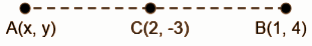2 = [x +1] / 2

4 – x = 1

x = 3

-3 = [y + 4] / 2

-6 – y = 4

y = -10

The coordinates of A are (3, -10).

Question 8: Find the area of the triangle, whose vertices are (-5, -1), (3, -5), (5, 2).

Solution:

Area = [1 / 2] [x1 (y2 – y3) + x2 (y3 – y1) + x3 (y1 – y2)]

= [1 / 2] [-5 * (-5 -2) + 3 (2 + 1) + 5 (-1 + 5)]

= [1 / 2] [35 + 9 + 20]

= [1 / 2] * 64

= 32 cm2

SECTION – II

[4 * 1 = 4]

Answer ANY 4 of the following six questions.

Question 9: Expand log 15.

Solution:

log 15 = log5 3

= log5 log3

Question 10: Write roster and set builder form of “The set of all-natural numbers, which divide 42”.

Solution:

(i) x = {1, 2, 3, 6, 7}

(ii) x = {x : x = 1, 2, 3, 6, 7}

Question 11: If p(t) = t3 – 1, find the values of p (1), p (–2).

Solution:

p(1) = 13 – 1 = 0

p(-2) = -23 – 1 = -8 – 1 = -9

Question 12: Formulate a pair of linear equations in two variables “5 pencils and 7 pens together cost Rs. 50, whereas 7 pencils and 5 pens together cost Rs. 46”.

Solution:

Let pencil = x

pen = y

5x + 7y = 50 * 5

7x + 5y = 46 * 7

25x + 35y = 250

49x + 35y = 322

______________

24x = 72

x = 42 / 24

x = 3

Pencil = 3

y = [46 – 7x] / 5

= [46 – 7 * 3] / 5

= 25 / 5

= 5

y = 5

Pen = 5

Question 13: If b2 – 4ac ≥ 0, then write the roots of a quadratic equation ax2 + bx + c = 0.

Solution:

Real, equal and distinct.

Question 14: Write the formula for the sum of first ‘n’ positive integers.

Solution:

n [n + 1] / 2

SECTION – III

[4 * 4 = 16]

Answer ANY 4 questions, choosing atleast two from each of the following groups.

GROUP – A

Question 15: Prove that √5 is irrational by the method of Contradiction.

Solution:

Say, √5 is a rational number.

∴ It can be expressed in the form p / q where p,q are co-prime integers.

⇒√5 = p / q

⇒ 5 = p² / q² {Squaring both the sides}

⇒ 5q² = p² — (1)

⇒ p² is a multiple of 5. {Euclid’s Division Lemma}

⇒ p is also a multiple of 5. {Fundamental Theorem of arithmetic}

⇒ p = 5m

⇒ p² = 25m² — (2)

From equations (1) and (2), we get,

5q² = 25m²

⇒ q² = 5m²

⇒ q² is a multiple of 5. {Euclid’s Division Lemma}

⇒ q is a multiple of 5.{Fundamental Theorem of Arithmetic}

Hence, p,q have a common factor 5.

This contradicts that they are co-primes.

Therefore, p / q is not a rational number.

So, √5 is an irrational number.

Question 16: If A = {3, 6, 9, 12, 15, 18, 21}; B = {4, 8, 12, 16, 20}; C = {2, 4, 6, 8, 10, 12, 14, 16}; D = {5, 10, 15, 20}; find

(i) A – B

(ii) B – A

(iii) C – A

(iv) D – A

(v) B – C

(vi) B – D

(vii) C – B

(viii) D – B

Solution:

(i) A – B = {3, 6, 9, 15, 18, 21}

(ii) B – A = {4, 8, 16, 20}

(iii) C – A = {2, 4, 8, 10, 14, 16}

(iv) D – A = {5, 10, 20}

(v) B – C = {20}

(vi) B – D = {4, 8, 12, 16}

(vii) C – B = {2, 6, 10, 14}

(viii) D – B = {5, 10, 15}

Question 17: Verify that 1, -1 and -3 are the zeroes of the cubic polynomial x3 + 3x2 – x – 3 and check the relationship between zeroes and the coefficient.

Solution:

To verify roots, put root inside equations x3 + 3x2 – x – 3

Root 1 ⟶ 13 + 3(1)2 – 1 – 3 = 1 + 3 – 1 – 3 = 0

Root (-1) = -13 + 3 (-1)2 + 1 – 3 = – 1 + 3 + 1 – 3 = 0

Root (-3) = (-3)3 + 3 (-3)2 – (-3) – 3 = – 27 + 27 + 3 – 3 = 0

Sum of root = -b / a = -3 / 1 = -3

From roots = (1 – 1 – 3) = –3

Product of roots = -d / a = 3 / 1 = 3

From roots = 1 * -1 * -3 = 3

Product of roots = pq + qr + rp = c / a = -1 / 1 = -1

From roots = 1 * –1 + –1 * –3 + –3 * 1 = – 1 + 3 – 3 = –1

Hence verified.

Question 18: Find the root of equation 2x2 – x – 4 = 0 by the method of completing the square.

Solution:

2𝑥2 − 1𝑥 − 4 = 0

𝑎 ≠ 1 so divide through by 2

[2 / 2] 𝑥2 − [1 / 2] 𝑥 − [4 / 2] = 0 / 2

𝑥2 − [1 / 2] 𝑥 − 2 = 0

Keep 𝑥 terms on the left and move the constant to the right side by adding it on both sides

𝑥2 − [1 / 2] 𝑥 = 2

Take half of the 𝑥 term and square it

{[−1 / 2] ⋅ [1 / 2]}2 = 1 / 16

Add the result to both sides

𝑥2 − [1 / 2] 𝑥 + [1 / 16] = 2 + [1 / 16]

Rewrite the perfect square on the left

(𝑥 − (1 / 4))2 = 2 + [1 / 16]

Combine terms on the right

(𝑥 − [1 / 4])2 = 33 / 16

Take the square root of both sides

𝑥 − [1 / 4] = ± √33 / 16

Simplify the Radical term (0):

𝑥 − [1 / 4] = ± √33 / 4

Isolate the x on the left side and solve for x (1)

𝑥 = [1 / 4] ± √33 / 4

𝑥 = [1 / 4] + √33 / 4

𝑥 = [1 / 4] − √33 / 4

GROUP – B

Question 19: Solve the equations  / [x – 1] +  / [y – 2] and  / [x – 1] –  / [y – 2] = 1.

Solution:

 / [x – 1] +  / [y – 2] — 1

 / [x – 1] –  / [y – 2] = 1 — 2

let assume, 1 / [x – 1] = u, 1 / [y – 2] = v

15 * u = 3 * v = 6 —) 3

Add equations 2 and 3

21* u = 7

u = (1 / 3)

x – 1 = 3

x = 4

b = 1 / 3

y – 2 = 3

y = 5

Question 20: A fraction becomes 4 / 5 if 1 is added to both numerator and denominator. If, however, 5 is subtracted from both numerator and denominator, the fraction becomes 1 / 2. What is the fraction?

Solution:

Let the fraction be p / q.

The fraction will become 4 / 5 if 1 is added to numerator and denominator

So,

4 / 5 = (p + 1) / (q + 1)

4q + 4 = 5p + 5

(p – 5) / (q – 5) = 1 / 2

2p – 10 = q – 5

q = 2p – 5

4(2p – 5) + 4 = 5p + 5

8p – 20 + 4 = 5p + 5

3p = 21

p = 7

Therefore q = 2 * 7 – 5

q = 9

So the fraction is 7/9.

Question 21: The sum of the 4th and 8th terms of an A.P is 24 and the sum of the 6th and 10th terms is 44. Find the first three terms of the A.P.

Solution:

Let the first term of an AP = a and the common difference of the given AP = d.

an = a + (n − 1) d

a4 = a + (4 − 1) d

a4 = a + 3d

Similarly,

a8 = a + 7d

a6 = a + 5d

a10 = a + 9d

The sum of 4th and 8th term = 24

a4 + a8 = 24

a + 3d + a + 7d = 24

2a + 10d = 24

a + 5d = 12……………….. (i)

The sum of 6th and 10th term = 44

a6 + a10 = 44

a + 5d + a + 9d = 44

2a + 14d = 44

a + 7d = 22 ………………….(ii)

Solving (i) and (ii),

a + 7d = 22

a + 5d = 12

2d = 10

d = 10 / 2

d = 5

From equation (i), we get,

a + 5d = 12

a + 5 (5) = 12

a + 25 = 12

a = −13

a2 = a + d = − 13 + 5 = −8

a3 = a2 + d = − 8 + 5 = −3

Question 22: Prove that the points (-7, -3), (5, 10), (15, 8) and (3, -5) taken in order are the vertices of a parallelogram.

Solution: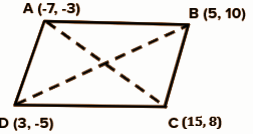Let say ABCD be the points of the parallelogram.

To prove: Correct order of vertices, diagonals bisect each other in the parallelogram. Hence point O must be some from A and C.

C = [-7 + 25] / 2, [-3 + 8] / 2

= [4, (5 / 2)]

From B and D

C = [-5 + 3] / 2, [10 – 5] / 2

= [4, (5 / 2)]

Hence, both are correct.

SECTION – IV

(1 * 5 = 5)

Answer ANY ONE question from the following.

Question 23: Draw the graph of y = x2 – x – 6 and find zeroes. Justify the answer.

Solution:

Clearly the graph of y = x2 − x − 6 cut the x-axis at x = −2, 3.

⇒x = −2, 3 are zeros of y = x2 − x − 6.

Justification:

when x = −2 ; y = 4 + 2 − 6 = 0

when x = 3 ; y = 9 − 3 − 6 = 0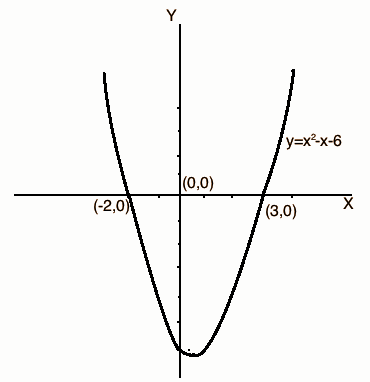Question 24: Solve the pair linear equations graphically.

2x + y – 6 = 0

4x – 2y – 4 = 0

Solution: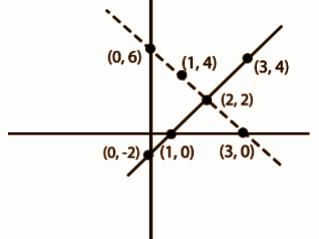Hence, the graph solution is (2,2).